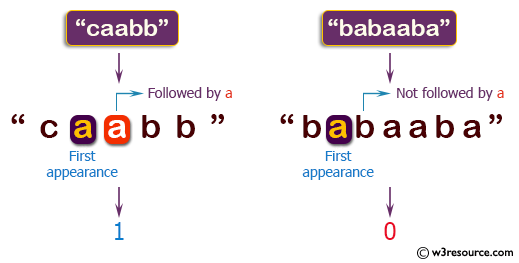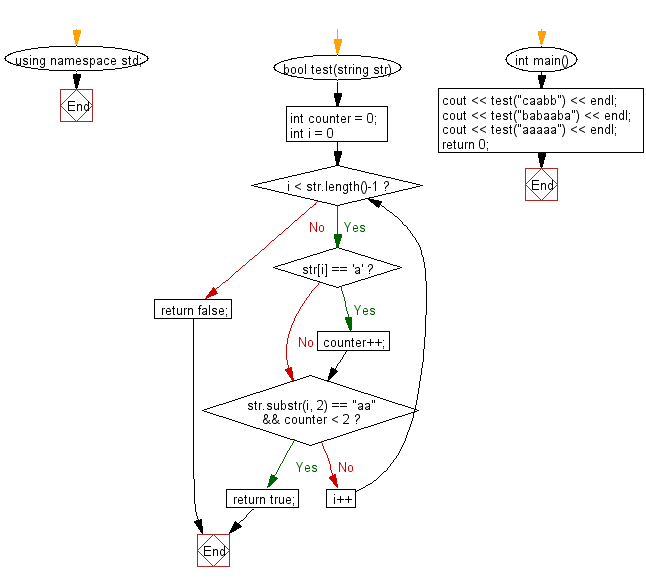﻿ C++ Exercises: Check if the first appearance of 'a' in a given string is immediately followed by another 'a' - w3resource# C++ Exercises: Check if the first appearance of 'a' in a given string is immediately followed by another 'a'

## C++ Basic Algorithm: Exercise-27 with Solution

Write a C++ program to check if the first appearance of "a" in a given string is immediately followed by another "a".

Sample Solution:

C++ Code :

``````#include <iostream>
using namespace std;

bool test(string str)
{
int counter = 0;
for (int i = 0; i < str.length()-1; i++)
{
if (str[i] == 'a') counter++;
if(str.substr(i, 2) == "aa" && counter < 2)
return true;
}
return false;
}

int main()
{
cout << test("caabb") << endl;
cout << test("babaaba") << endl;
cout << test("aaaaa") << endl;
return 0;
}
``````

Sample Output:

```1
0
1
```

Pictorial Presentation:Flowchart:C++ Code Editor:

Contribute your code and comments through Disqus.

What is the difficulty level of this exercise?

﻿